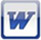THE education site for computer science and ICT

Numbers: Binary

Converting denary numbers to binary numbers: workbook

It has been assumed that students know how to convert denary to binary. This workbook is to consolidate those techniques.

It might be worth demonstrating how to answer the first question so that students understand how to use the workbook

(subscription only)Converting binary numbers to denary numbers: workbook

It has been assumed that students know how to convert binary to denary. This workbook is to consolidate those techniques.

It might be worth demonstrating how to answer the first question so that students understand how to use the workbook

(subscription only)It has been assumed that students know how to add binary numbers. This workbook is to consolidate those techniques.

It might be worth demonstrating how to answer the first question so that students understand how to use the workbook

(subscription only)